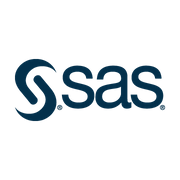39,647 recent views
Shareable Certificate
Earn a Certificate upon completion
100% online
Start instantly and learn at your own schedule.
Intermediate Level
Approx. 21 hours to complete
English
Shareable Certificate
Earn a Certificate upon completion
100% online
Start instantly and learn at your own schedule.
Intermediate Level
Approx. 21 hours to complete
English

### Offered byWeek
1

## Week 1

1 hour to complete

## Course Overview and Data Setup

1 hour to complete
2 videos (Total 13 min), 5 readings
2 videos
Demo: Exploring Ames Housing Data10m
Learner Prerequisites1m
Choosing and Setting Up SAS Software for this Course10m
Follow These Instructions to Set Up Data for This Course (REQUIRED)30m
Completing Demos and Practices10m
Using Forums and Getting Help10m
3 hours to complete

## Introduction and Review of Concepts

3 hours to complete
17 videos (Total 41 min), 2 readings, 9 quizzes
17 videos
Statistical Modeling: Types of Variables1m
Overview of Models3m
Explanatory versus Predictive Modeling1m
Population Parameters and Sample Statistics1m
Normal (Gaussian) Distribution2m
Standard Error of the Mean51s
Confidence Intervals2m
Statistical Hypothesis Test4m
p-Value: Effect Size and Sample Size Influence3m
Scenario48s
Performing a t Test4m
Demo: Performing a One-Sample t Test Using PROC TTEST3m
Scenario1m
Assumptions for the Two-Sample t Test2m
Testing for Equal and Unequal Variances2m
Demo: Performing a Two-Sample t Test Using PROC TTEST4m
Parameters and Statistics10m
Normal Distribution10m
9 practice exercises
Question 1.015m
Question 1.025m
Question 1.035m
Question 1.045m
Question 1.055m
Practice - Using PROC TTEST to Perform a One-Sample t Test20m
Question 1.065m
Practice - Using PROC TTEST to Compare Groups20m
Introduction and Review of Concepts30m
Week
2

## Week 2

4 hours to complete

## ANOVA and Regression

4 hours to complete
29 videos (Total 70 min), 2 readings, 14 quizzes
29 videos
Scenario58s
Identifying Associations in ANOVA with Box Plots1m
Demo: Exploring Associations Using PROC SGPLOT1m
Identifying Associations in Linear Regression with Scatter Plots1m
Demo: Exploring Associations Using PROC SGSCATTER2m
Scenario56s
The ANOVA Hypothesis1m
Partitioning Variability in ANOVA2m
Coefficient of Determination1m
F Statistic and Critical Values1m
The ANOVA Model2m
Demo: Performing a One-Way ANOVA Using PROC GLM6m
Scenario49s
Multiple Comparison Methods2m
Tukey's and Dunnett's Multiple Comparison Methods1m
Diffograms and Control Plots1m
Demo: Performing a Post Hoc Pairwise Comparison Using PROC GLM6m
Scenario53s
Using Correlation to Measure Relationships between Continuous Variables1m
Hypothesis Testing for a Correlation1m
Avoiding Common Errors When Interpreting Correlations5m
Demo: Producing Correlation Statistics and Scatter Plots Using PROC CORR6m
Scenario45s
The Simple Linear Regression Model1m
How SAS Performs Simple Linear Regression1m
Comparing the Regression Model to a Baseline Model2m
Hypothesis Testing and Assumptions for Linear Regression1m
Demo: Performing Simple Linear Regression Using PROC REG7m
What Does a CLASS Statement Do?10m
Correlation Analysis and Model Building10m
14 practice exercises
Question 2.015m
Question 2.025m
Question 2.035m
Question 2.045m
Practice - Performing a One-Way ANOVA20m
Question 2.055m
Question 2.065m
Practice - Using PROC GLM to Perform Post Hoc Parwise Comparisons20m
Question 2.075m
Question 2.085m
Practice - Describing the Relationship between Continuous Variables20m
Question 2.095m
Practice - Using PROC REG to Fit a Simple Linear Regression Model20m
ANOVA and Regression30m
Week
3

## Week 3

2 hours to complete

## More Complex Linear Models

2 hours to complete
13 videos (Total 43 min), 1 reading, 5 quizzes
13 videos
Scenario1m
Applying the Two-Way ANOVA Model3m
Demo: Performing a Two-Way ANOVA Using PROC GLM7m
Interactions3m
Demo: Performing a Two-Way ANOVA With an Interaction Using PROC GLM5m
Demo: Performing Post-Processing Analysis Using PROC PLM4m
Scenario44s
The Multiple Linear Regression Model2m
Hypothesis Testing for Multiple Regression1m
Multiple Linear Regression versus Simple Linear Regression2m
Demo: Fitting a Multiple Linear Regression Model Using PROC REG7m
The STORE Statement10m
5 practice exercises
Question 3.015m
Practice - Performing a Two-Way ANOVA Using PROC GLM20m
Question 3.025m
Practice - Performing Multiple Regression Using PROC REG20m
More Complex Linear Models30m
2 hours to complete

## Model Building and Effect Selection

2 hours to complete
11 videos (Total 28 min), 3 readings, 4 quizzes
11 videos
Scenario1m
Approaches to Selecting Models2m
The All-Possible Regressions Approach to Model Building1m
The Stepwise Selection Approach to Model Building3m
Interpreting p-Values and Parameter Estimates2m
Demo: Performing Stepwise Regression Using PROC GLMSELECT7m
Scenario37s
Information Criteria2m
Demo: Performing Model Selection Using PROC GLMSELECT5m
Activity - Optional Stepwise Selection Method Code10m
Information Criteria Penalty Components10m
All-Possible Selection
4 practice exercises
Question 4.015m
Practice - Using PROC GLMSELECT to Perform Stepwise Selection20m
Practice - Using PROC GLMSELECT to Perform Other Model Selection Techniques20m
Model Building and Effect Selection20m
Week
4

## Week 4

3 hours to complete

## Model Post-Fitting for Inference

3 hours to complete
18 videos (Total 46 min)
18 videos
Scenario40s
Assumptions for Regression2m
Verifying Assumptions Using Residual Plots3m
Demo: Examining Residual Plots Using PROC REG5m
Scenario47s
Identifying Influential Observations1m
Checking for Outliers with STUDENT Residuals1m
Checking for Influential Observations2m
Detecting Influential Observations with DFBETAS1m
Demo: Looking for Influential Observations Using PROC GLMSELECT and PROC REG5m
Demo: Examining the Influential Observations Using PROC PRINT6m
Handling Influential Observations1m
Scenario38s
Exploring Collinearity1m
Visualizing Collinearity2m
Demo: Calculating Collinearity Diagnostics Using PROC REG5m
Using an Effective Modeling Cycle1m
7 practice exercises
Practice: Using PROC REG to Examine Residuals20m
Question 5.015m
Practice: Using PROC REG to Generate Potential Outliers20m
Question 5.025m
Question 5.035m
Practice: Using PROC REG to Assess Collinearity20m
Model Post-Fitting for Inference30m
2 hours to complete

## Model Building for Scoring and Prediction

2 hours to complete
11 videos (Total 27 min), 1 reading, 4 quizzes
11 videos
Scenario27s
Predictive Modeling Terminology1m
Model Complexity51s
Building a Predictive Model2m
Model Assessment and Selection1m
Demo: Building a Predictive Model Using PROC GLMSELECT10m
Scenario29s
Preparing for Scoring1m
Methods of Scoring1m
Demo: Scoring Data Using PROC PLM4m
Partitioning a Data Set Using PROC GLMSELECT10m
4 practice exercises
Question 6.015m
Practice: Building a Predictive Model Using PROC GLMSELECT20m
Practice: Scoring Using the SCORE Statement in PROC GLMSELECT20m
Model Building for Scoring and Prediction30m